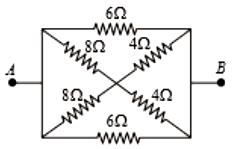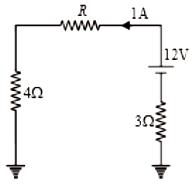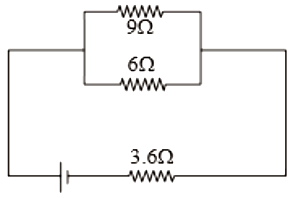A wire has resistance 24 $\Omega$. It is bent in the form of a circle. The effective resistance between the two point on the diameter of the circle is

(1) 6$\Omega$

(2) 4$\Omega$

(3) 3$\Omega$

(4) 12$\Omega$

Concept Questions :-

Combination of resistors
High Yielding Test Series + Question Bank - NEET 2020

Difficulty Level:

The drift velocity of the electrons in a current-carrying  metallic conductor is of the order of

1.

2.

3.

4.  ${10}^{-4}\mathrm{m}/\mathrm{s}$

Concept Questions :-

Current and current density
High Yielding Test Series + Question Bank - NEET 2020

Difficulty Level:

In the circuit shown in the figure, the effective resistance between A and B is1.  2$\mathrm{\Omega }$

2.  4$\mathrm{\Omega }$

3.  6$\mathrm{\Omega }$

4.  8$\mathrm{\Omega }$

Concept Questions :-

Combination of resistors
High Yielding Test Series + Question Bank - NEET 2020

Difficulty Level:

For the circuit shown in the figure, the value of R must be1.  3$\mathrm{\Omega }$

2.  4$\mathrm{\Omega }$

3.  5$\mathrm{\Omega }$

4.  6$\mathrm{\Omega }$

Concept Questions :-

Kirchoff's voltage law
High Yielding Test Series + Question Bank - NEET 2020

Difficulty Level:

If I be the current limit of a fuse wire of length l and radius r, then select the appropriate relation

(1)

(2)

(3)

(4)

Concept Questions :-

Heating effect of current
High Yielding Test Series + Question Bank - NEET 2020

Difficulty Level:

1.  220

2.  440

3.  310

4.  550

Concept Questions :-

Derivation of Ohm's law
High Yielding Test Series + Question Bank - NEET 2020

Difficulty Level:

1.

2.

3.

4.   $4$

High Yielding Test Series + Question Bank - NEET 2020

Difficulty Level:

The sensitivity of a potentiometer can be increased by

2.  decreasing the length of potentiometer wire

3.  decreasing the current in the potentiometer wire

4.  all of these

Concept Questions :-

Meter bridge and potentiometer
High Yielding Test Series + Question Bank - NEET 2020

Difficulty Level:1.  36V

2.  18V

3.  9V

4.  Zero

Concept Questions :-

Heating effect of current
High Yielding Test Series + Question Bank - NEET 2020

Difficulty Level:

A current of 1 mA is flowing through a copper wire. How many electrons will pass a given point in one second

1.

2.

3.

4.

Concept Questions :-

Current and current density